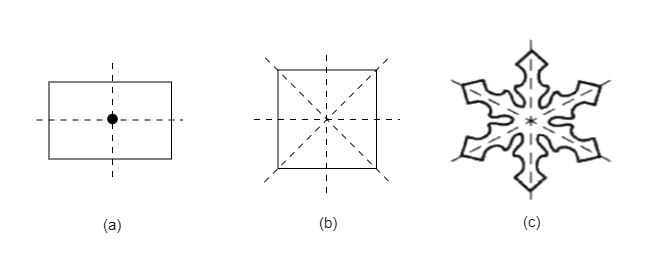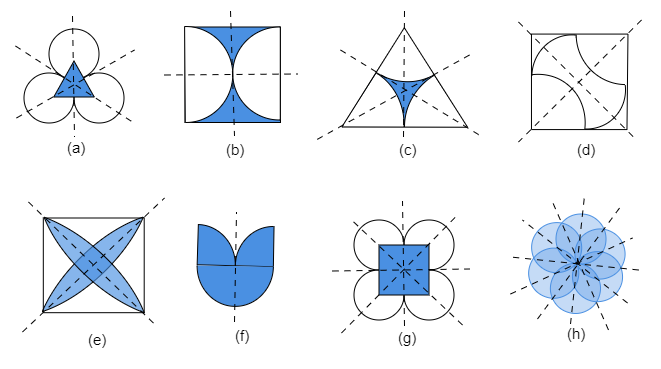# The following figures have more than one line of symmetry. Such figures are said to have multiple lines of symmetry.Identify multiple lines of symmetry

Given: Some figures have more than one line of symmetry as shown in the given figures. Such figures are said to have multiple lines of symmetry.

To do: To identify multiple lines of symmetry, if any, in each of the given figures.

Solution: In order to check the symmetry and find their lines of symmetry, we will draw several lines passing through the centre point in each shape.$(a)$.It has three lines of symmetry.

$(b)$.It has two lines of symmetry.

$(c)$.It has three lines of symmetry.

$(d)$.It has two lines of symmetry.

$(e)$.It has four lines of symmetry.

$(f)$.It has one line of symmetry.

$(g)$.It has four lines of symmetry.

$(h)$.It has six lines of symmetry.